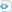# Posts tagged Visual Basic.Net

## Searching through all columns in datagridview

0

Assuming you are searching for the name John, the following procedure will search all existing columns regardless

 Source code```Dim x As Integer = 0
While x < DataGridView1.Rows.Count
Dim y As Integer = 0
While y < DataGridView1.Rows(x).Cells.Count
Dim c As DataGridViewCell = DataGridView1.Rows(x).Cells(y)
If Not c.Value Is DBNull.Value Or Nothing Then
If CType(c.Value, String) = "John" Then
MessageBox.Show("Found!")
End If
End If
End While
End While
MessageBox.Show("Search complete!")
End Sub```

## Filtering a datagridview

0

assuming you have a datagridview that has data in it.

you want to avoid recalling sql from the database & just want to filter the datagrid.

you can do the following, What you need (1 datagridview (data), 1 button (search), 1 textbox (string to search), 1 variable to precise the criteria your searching for)

 Source code```Dim findcrit as string
Dim dt As DataTable = Ds_Pos.Employees
Dim dv As New DataView(dt)

Dim _RowFilter As String = ""
Dim _FieldType = dt.Columns(findcrit).DataType.ToString

Select Case _FieldType
Case "System.Int32"
_RowFilter = "convert(" & findcrit & ", 'System.String') like '" & Me.TextBox1.Text & "'"
Case "System.Int64"
_RowFilter = "convert(" & findcrit & ", 'System.String') like '" & Me.TextBox1.Text & "'"
Case "System.Double"
_RowFilter = "convert(" & findcrit & ", 'System.String') like '" & Me.TextBox1.Text & "'"
Case "System.String"
_RowFilter = findcrit & " like '" & TextBox1.Text & "*'"
End Select
dv.RowFilter = _RowFilter
EmployeesDataGridView.DataSource = dv```

The cases are to cast the type of variable from any type to string because you can only use the method on string data types.

the idea is to move the content to a data table then filter then return it back to the datatable.

## Format input date in datagridview

0

A common problem faced in datagridview is how to format the date in the grid, here is a small way to do it:

Private Sub dataGridView1_CellParsing(ByVal sender As Object, ByVal e As DataGridViewCellParsingEventArgs)

If e.ColumnIndex = 0 Then

Dim [date] As String = DirectCast(e.Value, String)

Try

Dim month As String = [date].Substring(0, 2)

Dim day As String = [date].Substring(2, 2)

Dim year As String = [date].Substring(4, 4)

e.Value = New DateTime(Convert.ToInt32(year), Convert.ToInt32(month), Convert.ToInt32(day))

e.ParsingApplied = True
Catch ex As Exception

MessageBox.Show(“parsing error!”)

End Try

End If
End Sub

Go to Top